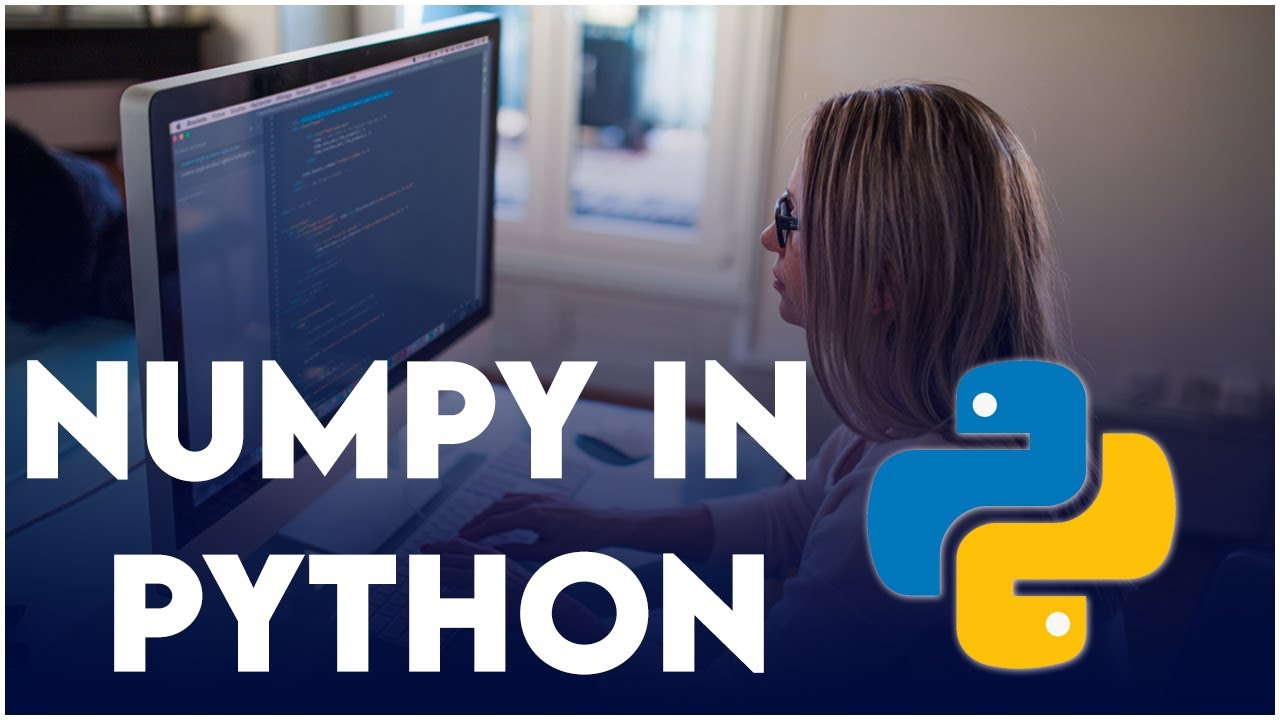# Python NumPy Tutorial for BeginnersNumPy, which stands for Numerical Python, is a library consisting of multidimensional array objects and a collection of routines for processing those arrays. Using NumPy, mathematical and logical operations on arrays can be performed.

NumPy, which stands for Numerical Python, is a library consisting of multidimensional array objects and a collection of routines for processing those arrays. Using NumPy, mathematical and logical operations on arrays can be performed. NumPy is the core library for scientific and numerical computing in Python. It provides high-performance multidimensional array object and tools for working with arrays. Now, let us get started and understand what NumPy actually is.

## Top Python Development Companies | Hire Python Developers

After analyzing clients and market requirements, TopDevelopers has come up with the list of the best Python service providers. These top-rated Python developers are widely appreciated for their professionalism in handling diverse projects. When...

## Python Hello World Program - Create & Run Your First Python Program in PyCharm

Python Hello World Program - Your first step towards Python world. Learn how to create the Hello World Python program in PyCharm.

## Is NumPy Faster Than Python

How much does Numpy’s linear algebra out-pace regular Python?

## Python NumPy Tutorial for Beginners

Learn the basics of the NumPy library in this tutorial for beginners. It provides background information on how NumPy works and how it compares to Python's Built-in lists. This video goes through how to write code with NumPy. It starts with the basics of creating arrays and then gets into more advanced stuff. The video covers creating arrays, indexing, math, statistics, reshaping, and more.

## Numpy isposinf() Function in Python

Numpy isposinf() function tests if an element positive infinity or not. If you need to test negative infinity then use numpy isneginf() in Python.